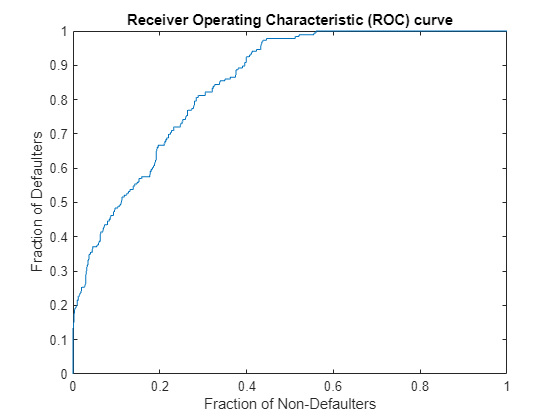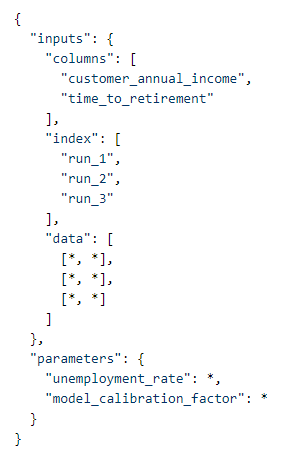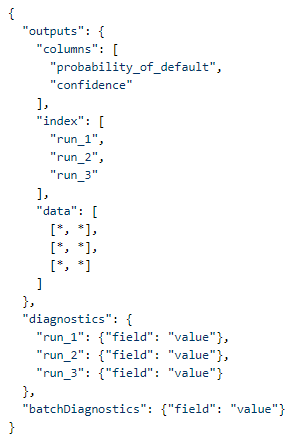# Validation of External Models

This example shows how to use MATLAB® model validation tools on models existing outside of MATLAB using Modelscape™.

Such a model could be implemented in Python®, R, SAS®, or it could be a MATLAB model deployed on a web service.

This example covers the use of external models from the point of view of a Model Validator or other model end user. Although the Validator does not need to know this, this example assumes that a model has been deployed to a microservice such as Flask (Python) or Plumber (R). The example also explains how such microservices and alternative interfaces should be implemented.

This example calls an external model from MATLAB to evaluate it with different inputs. The example then shows you how to implement the API for any external model so that it can be called from MATLAB.

### Call an External Model from MATLAB

This section shows you how to set up an interface and call an externally deployed model. This example uses a Python toy model although it could be implemented in any other programming language.

#### Setup the External Model

Use the Python code in the Appendix to set up mock data of a credit scoring model. This model adds noise to the input data, scales it, and returns the value as the output credit score of the applicant.

Run the script to make this "model" available in a test development server. The URL should be clearly visible in the output when the script is run. Copy it here if different from what is shown here:

`ModelURL = "http://172.26.249.170:5000/";`

In an actual model validation exercise, this information could be provided by the Model Developer as part of the validation request.

To set up a connection to this model, run the following:

`extModel = externalModelClient("RootURL", ModelURL)`
```extModel = ExternalModel with properties: InputNames: "income" ParameterNames: "weight" OutputNames: "score" ```

The model expects a single input called 'income' and a single parameter called 'weight', and returns a single output called 'score'.

The types and sizes of these inputs should be explained in model documentation, but you can also find this information in the `InputDefinition`, `ParameterDefinition` and `OutputDefinition` properties of `extModel.`

`extModel.InputDefinition`
```ans = struct with fields: sizes: [] dataType: [1×1 struct] ```
`extModel.InputDefinition.dataType`
```ans = struct with fields: name: "double" ```

Empty `sizes` property indicates that a scalar is expected.

### Evaluate the Model

Use the `evaluate` method of `ExternalModel` on your model`.` This method expects two inputs:

• The first input must be a table. Each row of the table must consist of the data for a single customer, or a single 'run'. The table is then a 'batch of runs'. The variable names of the table must match the `InputNames` shown by `ExternalModel`.

• The second input is a struct whose fields match the `ParameterNames` shown by `ExternalModel`. The values carried by this struct apply to all the runs in the batch. If the model has no parameters, omit this input.

The primary output is a table whose variable names match the `OutputNames` shown by `ExternalModel.` The rows correspond to the runs in the input batch. There may also be run-specific diagnostics consisting of one struct per run and a single batch diagnostic struct.

For your toy model, use random numbers in the range from 0 to 100,000 as customer incomes for the input data. For parameters, use a weight of 1.1.

```N = 1000; income = 1e5*rand(N,1); inputData = table(income, 'VariableNames',"income"); parameters = struct('weight', 1.1);```

```[modelScores, diagnostics, batchDiagnostics] = evaluate(extModel, inputData, parameters); head(modelScores)```
``` score ______ Row_1 110.66 Row_2 137.69 Row_3 52.155 Row_4 87.029 Row_5 73.207 Row_6 26.722 Row_7 36.671 Row_8 24.878 ```

The output is a table of the same size as the inputs. Not specifying the row names in input data defaults them to Row_1, Row2, and so on.

For this example, create a mock response variable by thresholding the income. Validate the scores of the above model against this response variable.

```defaultIndicators = income < 20000; % mocked-up response data aurocMetric = mrm.data.validation.pd.AUROC(defaultIndicators, modelScores.score); formatResult(aurocMetric)```
```ans = "Area under ROC curve is 0.8236" ```
`visualize(aurocMetric);`The toy model returns some diagnostics to illustrate their size and shape. The run-specific `diagnostics` are a single struct with a field for every run.

`diagnostics.Row_125`
```ans = struct with fields: noise: 1.7133e+04 ```

In this case, each struct records some 'noise' term that was used in the calculation of the model prediction. Batch diagnostics consist of a single struct carrying information shared across all runs, in this case, the elapsed valuation time at the server side.

`batchDiagnostics`
```batchDiagnostics = struct with fields: valuationTime: 0.0080 ```

#### Extra Arguments

Under the hood, `ExternalModel` talks to the model through a REST API. If necessary, the headers and HTTP options used for the corresponding message exchanges can be modified by passing extra `Headers` and `Options` arguments to `externalModelClient.` `Headers` should be of type `matlab.net.http.HeaderField` objects and `Options` should be of type `matlab.net.http.HTTPOptions`.

For example, extend the connection timeout to 20 seconds.

```options = matlab.net.http.HTTPOptions('ConnectTimeout',20); extModel = externalModelClient("RootURL", ModelURL, "Options", options) ```

### Implement an ExternalModel Interface

This part of the example explains how to implement an API for an external model to call it from MATLAB.

The `externalModelClient` function creates an object of type `mrm.validation.external.ExternalModel`. This object talks to the external model through a REST API, and it works with any model that implements the API below.

#### Endpoints

The API must implement two endpoints:

• `/signature` must accept a GET request and return a JSON string carrying the information about inputs, parameters and outputs.

• /`evaluate` must accept a POST request with inputs and parameters in a JSON format and must return a payload containing outputs, diagnostics, and batch diagnostics as a JSON string.

The status code for a successful response should be `200 OK`; note that this is the default in Flask, for example.

#### Evaluation Inputs

The `/evaluate` endpoint should accept a payload of the following format.The `columns` in `inputs` should list the input names, `index` should specify the row names, `data` should contain the actual input data one row at the time, and `parameters` should just record the parameters with their values. The asterisks indicate the values - for example doubles or strings.

Note that the `inputs` datum is compatible with the construction of Pandas DataFrames with `split` orientation; see the example implementation in the Appendix.

#### Response Formats

The `/signature` endpoint should return a payload of the following format:The /`evaluate` endpoint should return a payload in the following format:Note again that the `outputs` data is compatible with the JSON output of Pandas dataframes with `split` orientation.

See the sample code in the Appendix for an interface implementation used in the first part of this example.

### Work with Alternative APIs

This section explains how to make external models available to a Model Validator in MATLAB when the default API is either impossible or inconvenient to implement - for example when your organization already has a preferred REST API for evaluating models. For this, implement an API class that inherits from `mrm.validation.external.ExternalModelBase`. Package this implementation in a `+mrm/+validation/+external/` folder on the MATLAB path.

This custom API must populate the `InputNames`, `ParameterNames` and `OutputNames` properties shown to the Validator after an `externalModelClient` call. It must also implement the `evaluate` method which should take as inputs a table and a struct as in the default API `ExternalModel`. It is then the responsibility of the custom API to serialise the inputs, manage the REST API calls, and deserialise the outputs into tables and structs as shown above.

When a custom API has been implemented as, say, `mrm.validation.external.CustomAPI`, the Validator can initialize a connection to the model through this client by adding an `APIType` argument to the `externalModelClient` call.

```extModelNew = externalModelClient("APIType", "CustomAPI", "RootURL", ModelURL) ```

Any further arguments will also be passed through to `CustomAPI`.

```from flask import Flask, request, jsonify import pandas as pd import numpy as np import time toyModel = Flask(__name__) @toyModel.route('/evaluate', methods=['POST']) def calc(): start = time.time() data = request.get_json() inputData = data['inputs'] inputDF = pd.DataFrame(inputData['data'], columns=inputData['columns'], index=inputData['index']) parameters = data['parameters'] noise = np.random.uniform(low = -50000, high=50000, size=inputDF.shape) outDF = inputDF.rename(columns={'income':'score'}) outDF = outDF.add(noise) outDF = outDF.mul(parameters['weight']/1000) diagnostics = pd.DataFrame(noise, columns=["noise"], index=inputDF.index) end = time.time() batchDiagnostics = {'valuationTime' : end - start} output = {'outputs': outDF.to_json(orient='split'), 'diagnostics' : diagnostics.to_dict(orient='index'), 'batchDiagnostics' : batchDiagnostics} return output @toyModel.route('/signature', methods=['GET']) def getInputs(): outData = { 'inputs': [{"name": "income", "dataType": {"name": "double"},"sizes": []}], 'parameters': [{"name": "weight", "dataType": {"name": "double"}, "sizes": []}], 'outputs': [{"name": "score", "dataType": {"name": "double"}, "sizes": []}] } return(jsonify(outData)) if __name__ == '__main__': toyModel.run(debug=True, host='0.0.0.0') ```